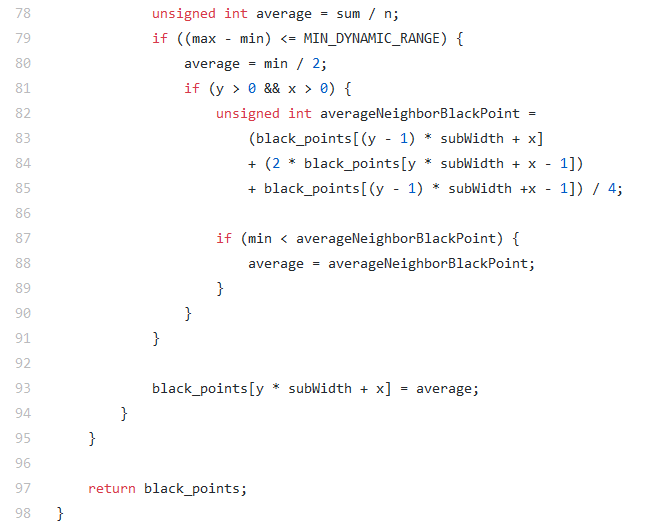### Project - Stage 2.2 - Project Optimize 2

Project Optimize Plan

#### The Second Algorithm Improvement

In general, multiply or divide by an integer can get the result by shifting it. The multiply and divide method - generate left-shifted code if multiply by 2n, and multiply by any other integer or divide by any number. The shift method is more efficient than the multiplication and division method.

binarize.cunsigned int averageNeighborBlackPoint = ... / 4  >>2